# Water Images Quiz I, Non Verbal Reasoning

## 25 Questions MCQ Test Non Verbal Reasoning | Water Images Quiz I, Non Verbal Reasoning

Description
Attempt Water Images Quiz I, Non Verbal Reasoning | 25 questions in 50 minutes | Mock test for LR preparation | Free important questions MCQ to study Non Verbal Reasoning for LR Exam | Download free PDF with solutions
QUESTION: 1

Solution:
QUESTION: 2

Solution:
QUESTION: 3

### Directions to Solve In each of the following questions, you are given a combination of alphabets and/or numbers followed by four alternatives (1), (2), (3) and (4). Choose the alternative which is closely resembles the water-image of the given combination. Question - Choose the alternative which is closely resembles the water-image of the given combination.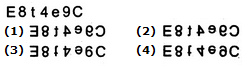Solution:
QUESTION: 4

In each of the following questions, you are given a combination of alphabets and/or numbers followed by four alternatives (1), (2), (3) and (4). Choose the alternative which is closely resembles the water-image of the given combination.

Question -

Choose the alternative which is closely resembles the water-image of the given combination.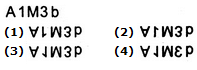Solution:
QUESTION: 5

In each of the following questions, you are given a combination of alphabets and/or numbers followed by four alternatives (1), (2), (3) and (4). Choose the alternative which is closely resembles the water-image of the given combination.

Question -

Choose the alternative which is closely resembles the water-image of the given combination.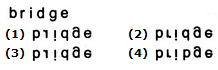Solution:
QUESTION: 6

In each of the following questions, you are given a combination of alphabets and/or numbers followed by four alternatives (1), (2), (3) and (4). Choose the alternative which is closely resembles the water-image of the given combination.

Question -

Choose the alternative which is closely resembles the water-image of the given combination.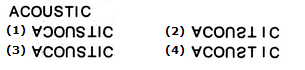Solution:
QUESTION: 7

In each of the following questions, you are given a combination of alphabets and/or numbers followed by four alternatives (1), (2), (3) and (4). Choose the alternative which is closely resembles the water-image of the given combination.

Question -

Choose the alternative which is closely resembles the water-image of the given combination.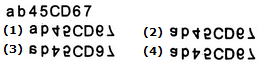Solution:
QUESTION: 8

In each of the following questions, you are given a combination of alphabets and/or numbers followed by four alternatives (1), (2), (3) and (4). Choose the alternative which is closely resembles the water-image of the given combination.

Question -

Choose the alternative which is closely resembles the water-image of the given combination.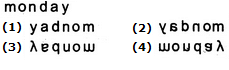Solution:
QUESTION: 9

In each of the following questions, you are given a combination of alphabets and/or numbers followed by four alternatives (1), (2), (3) and (4). Choose the alternative which is closely resembles the water-image of the given combination.

Question -

Choose the alternative which is closely resembles the water-image of the given combination.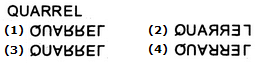Solution:
QUESTION: 10

In each of the following questions, you are given a combination of alphabets and/or numbers followed by four alternatives (1), (2), (3) and (4). Choose the alternative which is closely resembles the water-image of the given combination.

Question -

Choose the alternative which is closely resembles the water-image of the given combination.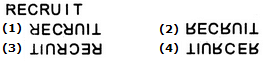Solution:
QUESTION: 11

In each of the following questions, you are given a combination of alphabets and/or numbers followed by four alternatives (1), (2), (3) and (4). Choose the alternative which is closely resembles the water-image of the given combination.

Question -

Choose the alternative which is closely resembles the water-image of the given combination.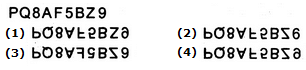Solution:
QUESTION: 12

In each of the following questions, you are given a combination of alphabets and/or numbers followed by four alternatives (1), (2), (3) and (4). Choose the alternative which is closely resembles the water-image of the given combination.

Question -

Choose the alternative which is closely resembles the water-image of the given combination.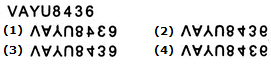Solution:
QUESTION: 13

In each of the following questions, you are given a combination of alphabets and/or numbers followed by four alternatives (1), (2), (3) and (4). Choose the alternative which is closely resembles the water-image of the given combination.

Question -

Choose the alternative which is closely resembles the water-image of the given combination.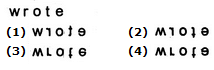Solution:
QUESTION: 14

In each of the following questions, you are given a combination of alphabets and/or numbers followed by four alternatives (1), (2), (3) and (4). Choose the alternative which is closely resembles the water-image of the given combination.

Question -

Choose the alternative which is closely resembles the water-image of the given combination.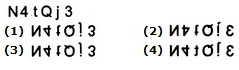Solution:
QUESTION: 15

In each of the following questions, you are given a combination of alphabets and/or numbers followed by four alternatives (1), (2), (3) and (4). Choose the alternative which is closely resembles the water-image of the given combination.

Question -

Choose the alternative which is closely resembles the water-image of the given combination.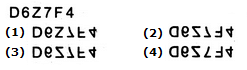Solution:
QUESTION: 16

In each of the following questions, you are given a combination of alphabets and/or numbers followed by four alternatives (1), (2), (3) and (4). Choose the alternative which is closely resembles the water-image of the given combination.

Question -

Choose the alternative which is closely resembles the water-image of the given combination.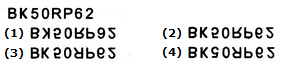Solution:
QUESTION: 17

In each of the following questions, you are given a combination of alphabets and/or numbers followed by four alternatives (1), (2), (3) and (4). Choose the alternative which is closely resembles the water-image of the given combination.

Question -

Choose the alternative which is closely resembles the water-image of the given combination.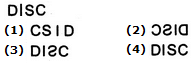Solution:
QUESTION: 18

In each of the following questions, you are given a combination of alphabets and/or numbers followed by four alternatives (1), (2), (3) and (4). Choose the alternative which is closely resembles the water-image of the given combination.

Question -

Choose the alternative which is closely resembles the water-image of the given combination.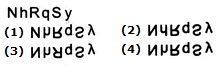Solution:
QUESTION: 19

In each of the following questions, you are given a combination of alphabets and/or numbers followed by four alternatives (1), (2), (3) and (4). Choose the alternative which is closely resembles the water-image of the given combination.

Question -

Choose the alternative which is closely resembles the water-image of the given combination.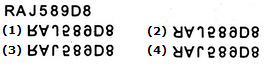Solution:
QUESTION: 20

In each of the following questions, you are given a combination of alphabets and/or numbers followed by four alternatives (1), (2), (3) and (4). Choose the alternative which is closely resembles the water-image of the given combination.

Question -

Choose the alternative which is closely resembles the water-image of the given combination.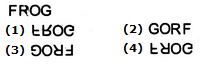Solution:
QUESTION: 21

In each of the following questions, you are given a combination of alphabets and/or numbers followed by four alternatives (1), (2), (3) and (4). Choose the alternative which is closely resembles the water-image of the given combination.

Question -

Choose the alternative which is closely resembles the water-image of the given combination.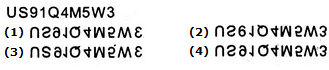Solution:
QUESTION: 22

In each of the following questions, you are given a combination of alphabets and/or numbers followed by four alternatives (1), (2), (3) and (4). Choose the alternative which is closely resembles the water-image of the given combination.

Question -

Choose the alternative which is closely resembles the water-image of the given combination.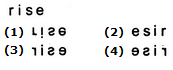Solution:
QUESTION: 23

In each of the following questions, you are given a combination of alphabets and/or numbers followed by four alternatives (1), (2), (3) and (4). Choose the alternative which is closely resembles the water-image of the given combination.

Question -

Choose the alternative which is closely resembles the water-image of the given combination.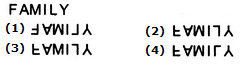Solution:
QUESTION: 24

In each of the following questions, you are given a combination of alphabets and/or numbers followed by four alternatives (1), (2), (3) and (4). Choose the alternative which is closely resembles the water-image of the given combination.

Question -

Choose the alternative which is closely resembles the water-image of the given combination.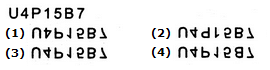Solution:
QUESTION: 25

In each of the following questions, you are given a combination of alphabets and/or numbers followed by four alternatives (1), (2), (3) and (4). Choose the alternative which is closely resembles the water-image of the given combination.

Question -

Choose the alternative which is closely resembles the water-image of the given combination.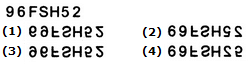Solution:Use Code STAYHOME200 and get INR 200 additional OFF Use Coupon Code